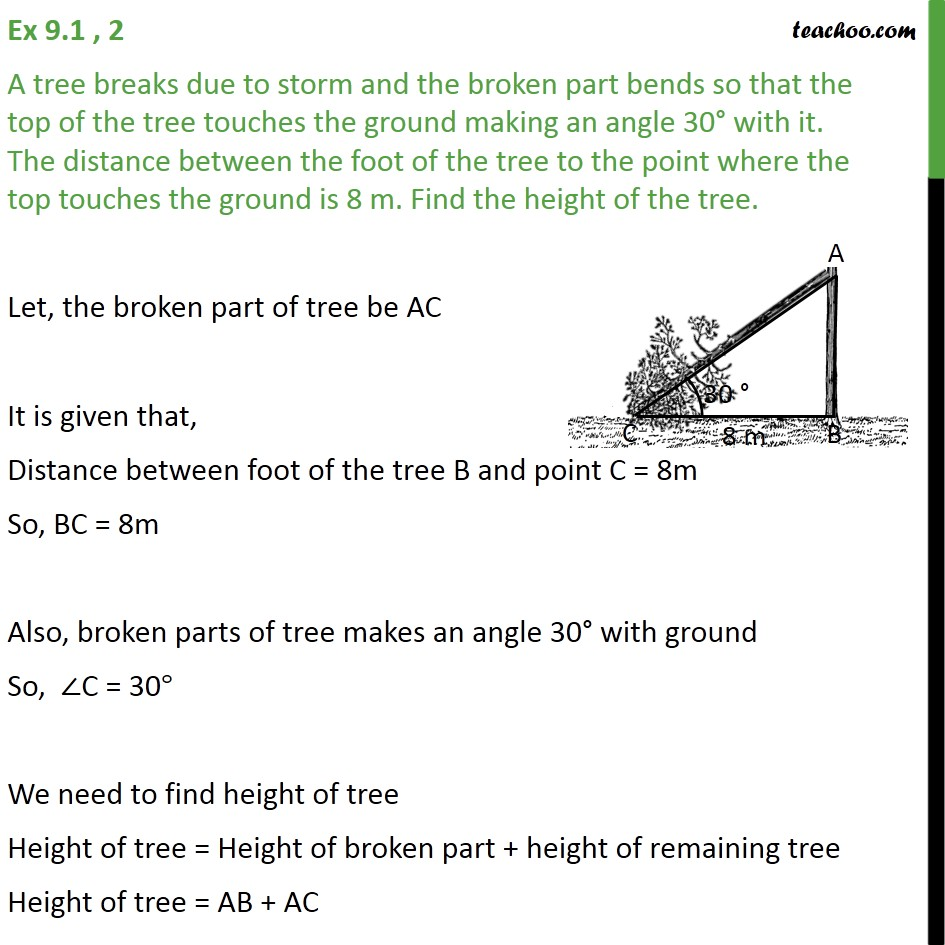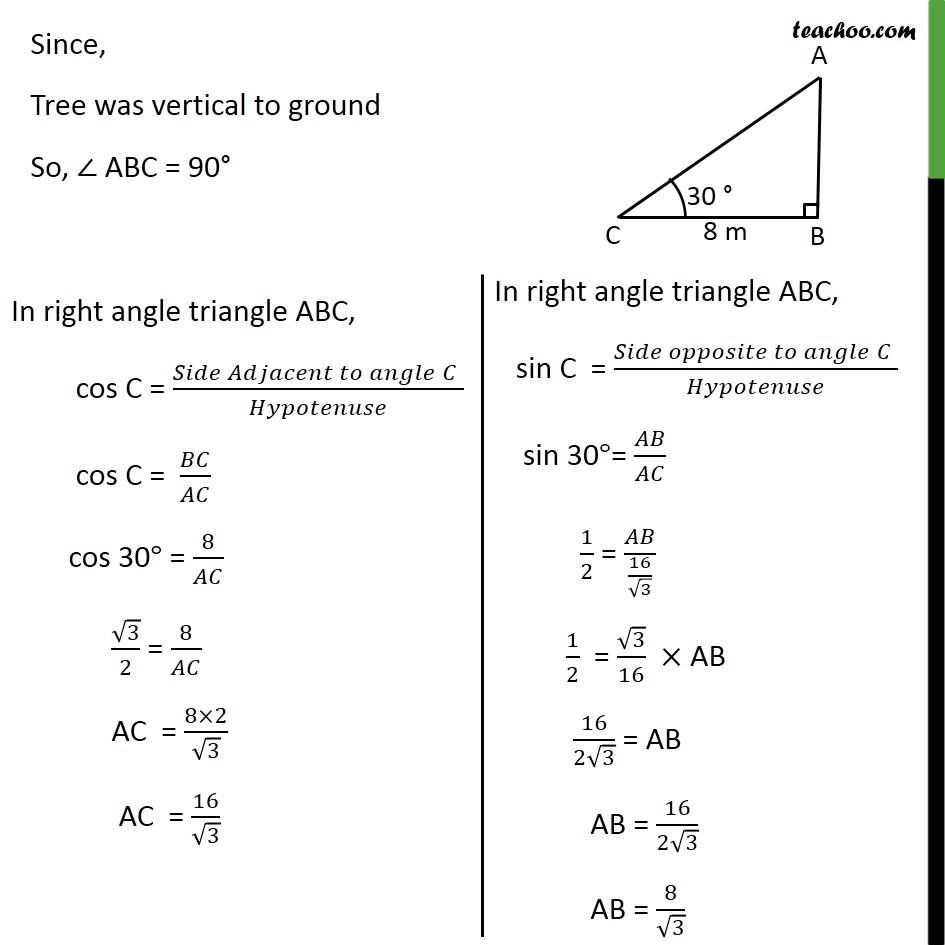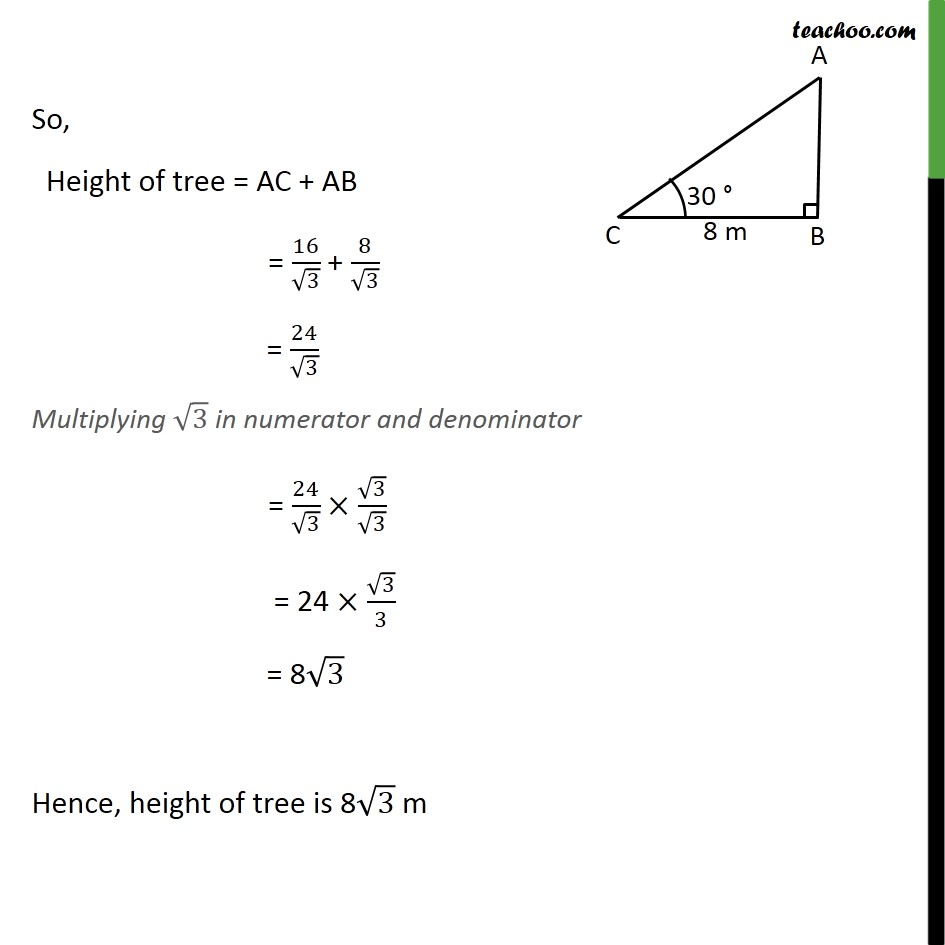1. Chapter 9 Class 10 Some Applications of Trigonometry (Term 2)
2. Concept wise
3. Questions easy to difficult

Transcript

Ex 9.1 , 2 A tree breaks due to storm and the broken part bends so that the top of the tree touches the ground making an angle 30° with it. The distance between the foot of the tree to the point where the top touches the ground is 8 m. Find the height of the tree. Let, the broken part of tree be AC It is given that, Distance between foot of the tree B and point C = 8m So, BC = 8m Also, broken parts of tree makes an angle 30° with ground So, ∠C = 30° We need to find height of tree Height of tree = Height of broken part + height of remaining tree Height of tree = AB + AC Since, Tree was vertical to ground So, ∠ ABC = 90° So, Height of tree = AC + AB = 16/√3 + 8/√3 = 24/√3 Multiplying √3 in numerator and denominator = 24/√3 × √3/√3 = 24 × √3/3 = 8√3 Hence, height of tree is 8√3 m